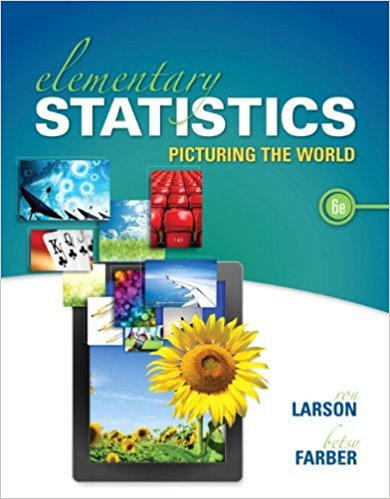# Individual Stock Price An individual stock is selected at## Problem 76E Chapter 3.1

Elementary Statistics: Picturing the World | 6th Edition

• 2901 Step-by-step solutions solved by professors and subject experts
• Get 24/7 help from StudySoup virtual teaching assistantsElementary Statistics: Picturing the World | 6th Edition

4 5 0 273 Reviews
15
1
Problem 76E

Individual Stock Price An individual stock is selected at random from the portfolio represented by the box-and-whisker plot shown. Find the probability that the stock price is (a) less than \$21, (b) between \$21 and \$50, and (c) \$30 or more.Step-by-Step Solution:

Step 1 of 3:

Given the portfolio represented by the box-and-whisker plot is given below,Our goal is:

a). We need to find the probability that the stock price less than 21.

b). We need to find the probability that the stock price between 21 and 50.

c). We need to find the probability that the stock price 30 or more.

a). From the above plot, the following information is

The minimum value is 12 and thepercentile is 21.

The median (50th percentile) is 30

Then the maximum value is 94 and the 75th percentile is 50.

From the given information we know that to understand the data, this means 25% of the data is between the minimum and thepercentile.

Then 25% of the data is between thepercentile and median.

Now we have to find the probability less than 21.

So It's no coincidence that this is thepercentile.

Here 25% of the data is below 21 so the probability is 25%.

Therefore, the probability that the stock price less than 21 is 25 percent.

Step 2 of 3:

b). From the above plot, 50 is thepercentile while 21 is thepercentile.

So this indicates that 50% of the data is between 21 and 50.

Then the probability that the stock price between 21 and 50 is

= 75% - 25%

= 50%

Therefore, the probability that the stock price between 21 and 50 is 50%.

Step 3 of 3

##### ISBN: 9780321911216

Unlock Textbook Solution

Individual Stock Price An individual stock is selected at

×
Get Full Access to Elementary Statistics: Picturing The World - 6th Edition - Chapter 3.1 - Problem 76e

Get Full Access to Elementary Statistics: Picturing The World - 6th Edition - Chapter 3.1 - Problem 76e

I don't want to reset my password

Need help? Contact support

Need an Account? Is not associated with an account
We're here to help